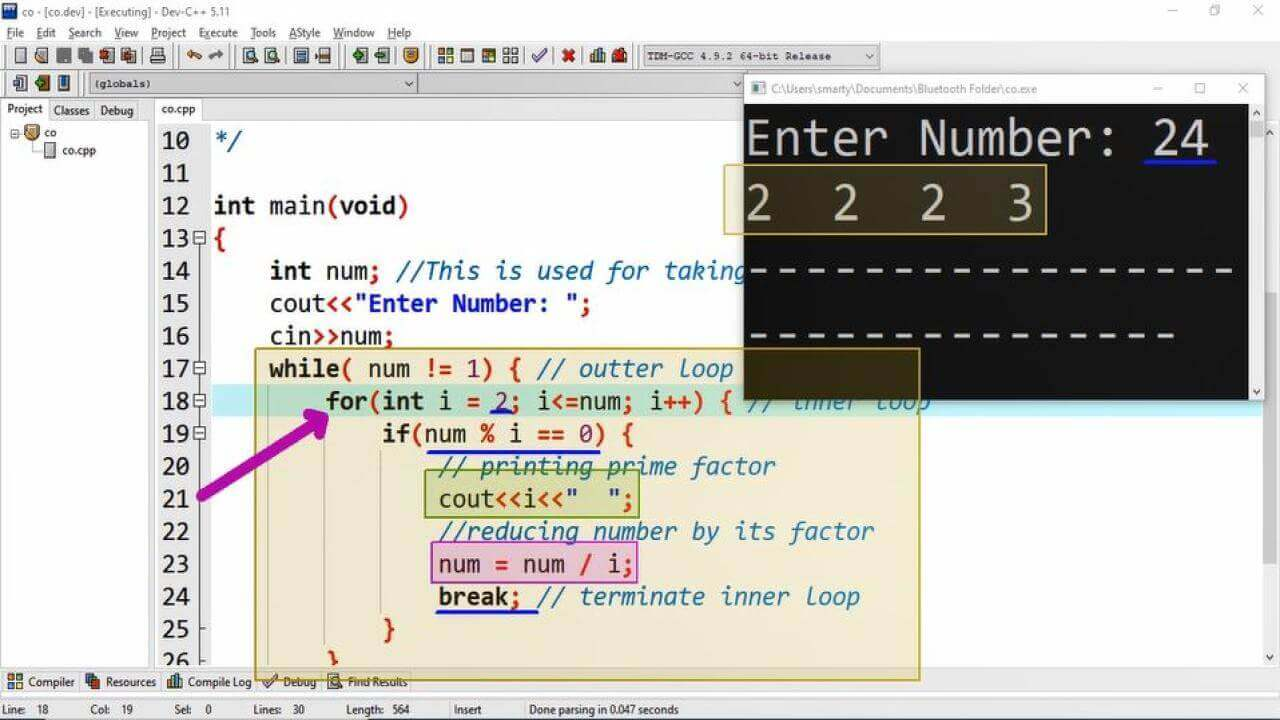# Program To Print Prime Factors in CPP

## Source Code:

#include <iostream>
using namespace std;
/*
Procedure to get Prime Factor
Number = 12
Prime Factors ?
12 = 2, 2, 3 => 2 x 2 x 3 = 12
Factors ?
12 = 1,  2, 3, 4, 6, 12
*/

int main(void)
{
int num; //This is used for taking input
cout<<"Enter Number: ";
cin>>num;
while( num != 1) { // outter loop
for(int i = 2; i<=num; i++) { // inner loop
if(num % i == 0) {
// printing prime factor
cout<<i<<"  ";
//reducing number by its factor
num = num / i;
break; // terminate inner loop
}
}
}
return 0;
}

## Output:## Working:

Every natural number is also multiplication of its prime factors. Prime factors can be obtained from a given number by dividing it from 2. 1 is not a prime number so that we start our iterations from 2.

In this program implementation we have used nested loop. The outer loop is while loop which will check eithe the number goes to 1 after frequently dividing it. And the inner loop is for loop , which is used to get prime factors of number. When any number in inner loop completely divide the number (menas its factor) then we print this number and terminte inner loop using break statement.

The logic behind termination is that when particular i (loop variable) divide number then after printing and dividing the number by this i. Next reduced number will not be divisible by upcoming values of counter variable i. So that to skip next unnecessary itterations of inner loop we use here break statement.

When the written outter loop completed all the prime factors of given number will be printed on the screen. We can multiply these prime factors to get the orignal number.

In body of inner loop we use if statement for checking whether the remaining number is completely divisible by the counter variable. If it completely divide then we will print the value of inner loop counter variable.